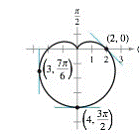Chapter 10.4, Problem 60E

Chapter
Section
Textbook Problem

# Finding Slopes of Tangent Lines In Exercises 63 and 64, find dy / dx and the slopes of the tangent lines shown on the graph of the polar equation. r = 2 ( 1 − sin θ )To determine

To Calculate:

The term dydx for equation r=2(1sinθ) and the slopes of tangent shown on graph of polar equation.

Explanation

Given:

The equation is r=2(1sinθ) and figure is shown as below:

Formula Used:

The formula is y=rsinθ

Calculation:

Consider the equation,

r=2(1sinθ)

Multiply the above equation both sides with sinθ,

rsinθ=2sinθ(1sinθ)

As it is known that  y=rsinθ substitute this value in above equation,

y=2sinθ2sin2θ

Now differentiate both sides with respect to θ

dydθ=2cosθ4sinθcosθ..(1)

In similarway multiply the given polar equation with cosθ,

rcosθ=2cosθ(1sinθ)

As it is known that x=rcosθ substitute this value in above equation,

x=2cosθ(1sinθ)

x=2cosθ2cosθsinθ

Now differentiate both sides with respect to θ,

dxdθ=2sinθ2cos2θ+2sin2θ

=22sinθ4cos

### Still sussing out bartleby?

Check out a sample textbook solution.

See a sample solution

#### The Solution to Your Study Problems

Bartleby provides explanations to thousands of textbook problems written by our experts, many with advanced degrees!

Get Started

#### Differentiate the function. y=x2+4x+3x

Single Variable Calculus: Early Transcendentals, Volume I

#### (71/2)4

Applied Calculus for the Managerial, Life, and Social Sciences: A Brief Approach

#### Graph y=x212x a. in the standard window b. in xmin=10,xmax=30;ymin=40,ymax=40

Mathematical Applications for the Management, Life, and Social Sciences